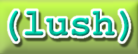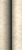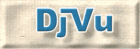# Energy-Based Models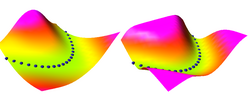• Time Period: September 2003 - present.
• Participants: Yann LeCun, Sumit Chopra, Raia Hadsell, Fu-Jie Huang, Marc'Aurelio Ranzato (Courant Institute/CBLL)

 Tutorials, Talks, and Videos

 Publications

• [LeCun et al 2006]. A Tutorial on Energy-Based Learning, in Bakir et al. (eds) "Predicting Structured Outputs", MIT Press 2006: a 60-page tutorial on energy-based learning, with an emphasis on structured-output models. The tutorial includes an annotated bibliography of discriminative learning, with a simple view of CRF, maximum-margin Markov nets, and graph transformer networks.
• [LeCun and Huang, 2005]. Loss Functions for Discriminative Training of Energy-Based Models. Proc. AI Stats 2005.
• [Osadchy, Miller, and LeCun, 2004] Synergistic Face Detection and Pose Estimation Proc. NIPS 2004. This paper uses an energy-based model methodology and contrastive loss function to detect faces and simultaneously estimate their pose.

 Description

Probabilistic graphical models associate a probability to each configuration of the relevant variables. Energy-based models (EBM) associate an energy to those configurations, eliminating the need for proper normalization of probability distributions. Making a decision (an inference) with an EBM consists in comparing the energies associated with various configurations of the variable to be predicted, and choosing the one with the smallest energy. Such systems must be trained discriminatively to associate low energies to the desired configurations and higher energies to undesired configurations. A wide variety of loss function can be used for this purpose. We give sufficient conditions that a loss function should satisfy so that its minimization will cause the system to approach to desired behavior.

An EBM can be seen as a scalar energy function of three (vector) variables E(Y,Z,X), parameterized by a set of trainable parameters W. X is the input, which is always observed (e.g. pixels from a camera), Y is the set of variables to be predicted (e.g. the label of the object in the input image), and Z is a set of latent variables that are never directly observed (e.g. the pose of the object).

Performing an inference consists in observing an X, and looking for the values of Z and Y that minimize the energy E(Y,Z,X).

Given a training set S={ (X1,Y1), (X2,Y2),....(Xp,Yp) }, training an EBM consists in "digging holes" in the energy surface at the training samples (Xi,Yi), and "building hills" everywhere else. This process will make the desired Yi become a minimum of the energy for the given Xi.

The main question is how to design a loss function so that minimizing this loss function with respect to the parameter vector W will have the effect of digging holes and building hills at the required places in the energy surface.

In the following, we demonstrate how various types of loss functions shape the energy surface. The task to learn is the square function Y=X^2. In the animations, the blue spheres indicate the location of training samples (a subset of the training samples). A good loss function will shape the energy surface so that the blue spheres are minima (over Y) of the energy for a given X.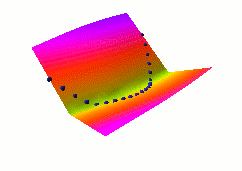Here, we demonstrate how "traditional" supervised learning applied to a traditional neural net (viewed as an EBM) shapes the energy surface. The EBM architecture is a traditional 2-layer neural net with 20 hidden units, followed by a cost module that measures the L1 distance between the network output and the desired output Y. The value of Y that minimizes the energy is simply equal to the neural net output. The loss function used here is simply the average squared energy over the training set. This is the traditional square loss used in conventional neural nets. With this architecture, the shape of the energy as a function of Y is constant (it's a V-shaped function), only the location of its minimum can change. Therefore, digging a hole at the right Y will automatically make the other values of Y have higher energies.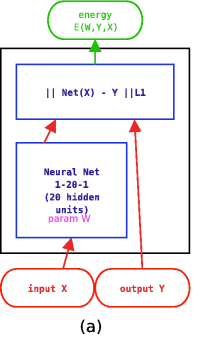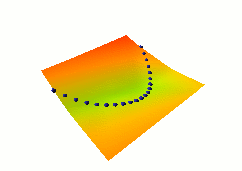In this second example, we use a different architecture, shown at right, where both X and Y are fed to neural nets. The outputs of the nets are fed to an L1 distance module. The loss function used here is again the average squared energy over the training set. The shape of the energy as a function of Y is no longer constant, but depends on the neural net on the right. A catastrophic collapse happens: the two neural nets are perfectly happy to simply ignore X and Y and produce identical, constant outputs (the flat green surface is the solution, the orange-ish surface is the random initial condition). The loss function pulls down on the blue spheres, but nothing is pulled up, and nothing prevents the energy surface from becoming flat. It does become flat. Hence the collapse.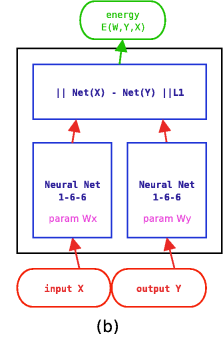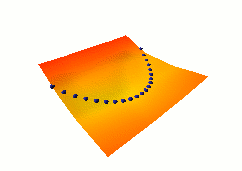In this third example, we use the same dual net architecture, but we use the so-called square-square generalized margin loss function which not only pulls down on the blue spheres, but also pulls up on points on the surface some distance away from the blue spheres. The point being pulled up for a given X is the Y with the lowest energy that is at least 0.3 away from the desired Y. This time, no collapse occurs, and the energy surface takes the right shape.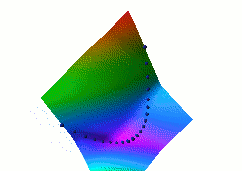In this fourth example, we use the same dual net architecture, trained with the negative-log-likelihood loss function. This loss pulls down on the blue spheres, and pulls up on all points of the surface, pulling harder on points with lower energy. This is the loss used to train traditional probabilistic models by maximizing the conditional likelihood of all the Yi given all the Xi. Again, no collapse occurs, but the computation is considerably more expensive than in the previous example, because we need to compute the integral over all values of Y of the derivative of the energy with respect to W (the derivative of the log partition function). Also, this loss function makes the energies of undesired Y grow without bounds, and it does not pull the energies of the blue spheres toward zero (only relative values matter)..